# Protractor

A geometric tool that contains a scale marked from zero to one hundred eighty degrees is called a protractor.

## Introduction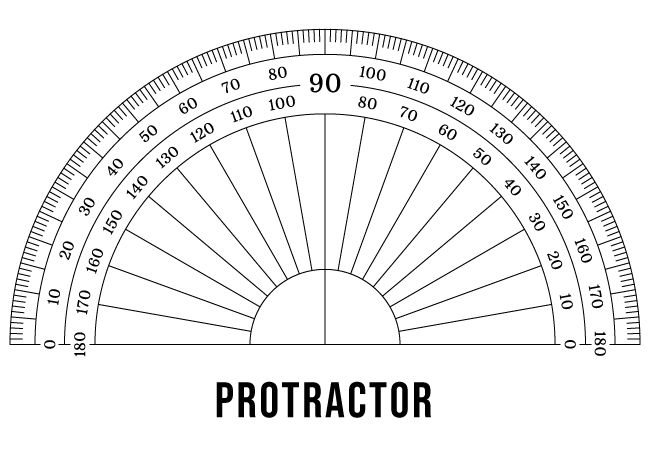A protractor is a basic geometric instrument and it is used to measure the angles in mathematics.

It is made of plastic or metal and it is actually made in semicircular shape but some protractors are made in circular shape too. However, a semicircular protractor is basically used while learning to measure the angles geometrically.

It contains two circular scales in degrees and each scale is marked from zero degrees to one hundred eighty degrees semi-circularly.

## Parts

A protractor contains three factors and they play vital role in measuring the angles in geometric mathematics.

### Baseline

A straight line, which is displayed in horizontal direction at the bottom of a protractor is called the baseline of the protractor. It seems the horizontal straight-line is a base of the protractor. Hence, it is considered as a baseline of the protractor.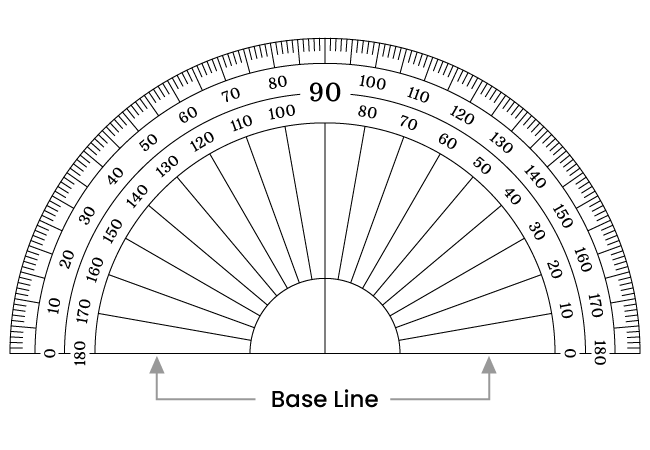The baseline of a protractor is bisected by a vertical line at its middle point. So, the two parts of the baseline are called by the following names in geometry.

1. Left-side baseline
2. Right-side baseline

Now, let’s learn the difference between them in detail.

##### Left side baseline

The part of the baseline, which is left side to the intersection point of the baseline and the vertical line is called the left side baseline.

##### Right side baseline

The part of the baseline, which is right side to the intersection point of the baseline and the perpendicular line is called the right side baseline.

### Origin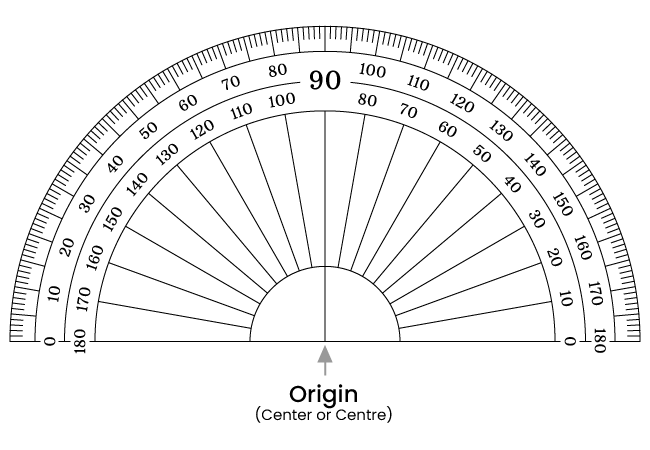A point where the baseline and a perpendicular line get intersected at the bottom of the protractor is called the origin of the protractor.

At the bottom of a protractor, the baseline is exactly bisected by a vertical line. Hence, the origin of the protractor is also called by the following two other names.

1. Center or Centre
2. Midpoint

Different people use different terminology for expressing it but these three names are commonly used to represent the origin of a protractor.

### Degree scale

There are three notable factors in degree scale of a protractor.

1. Degree marks
2. Inner scale or Anticlockwise degree scale
3. Outer scale or Clockwise degree scale

Now, let’s learn about them in detail with understandable graphical representations.

##### Degree marks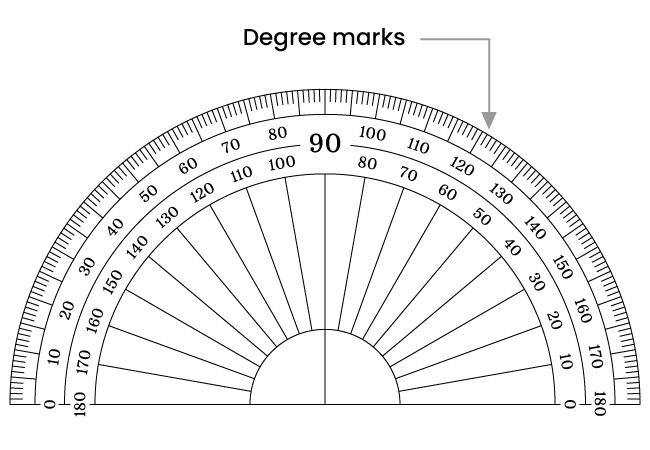Geometrically, the angle inside a semicircle is $180^\circ$. Hence, a semicircular protractor represents an angle of $180$ degrees, which is divided into one hundred eighty equal parts by marking with small line segments at the circular edge of the protractor.

For our convenience, the one hundred eighty line segments are marked in small, medium and large sizes. Every one degree ($1^\circ$) is denoted by a small size line segment, every five degrees ($5^\circ$) is represented by a medium size line segment and every ten degrees ($10^\circ$) is denoted by a large size line segment.

##### Inner degree scale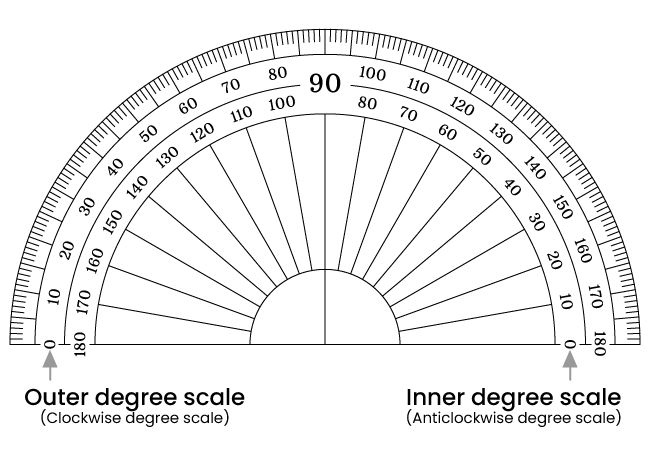At right side, the semicircular degree scale is denoted by the numbers from $0$ to $180$ in anticlockwise direction. Hence, it is called the anticlockwise degree scale. It is actually displayed below to another scale. So, it is also called the inner degree scale.

##### Outer degree scale

At left side, the semicircular degree scale is denoted by the numbers from $0$ to $180$ in clockwise direction. Hence, it is called the clockwise degree scale. It is actually displayed above to another scale. Hence, it is also called the outer degree scale.

Latest Math Topics
Jun 26, 2023
Jun 23, 2023

Latest Math Problems
Jul 01, 2023
Jun 25, 2023
###### Math Questions

The math problems with solutions to learn how to solve a problem.

Learn solutions

Practice now

###### Math Videos

The math videos tutorials with visual graphics to learn every concept.

Watch now

###### Subscribe us

Get the latest math updates from the Math Doubts by subscribing us.